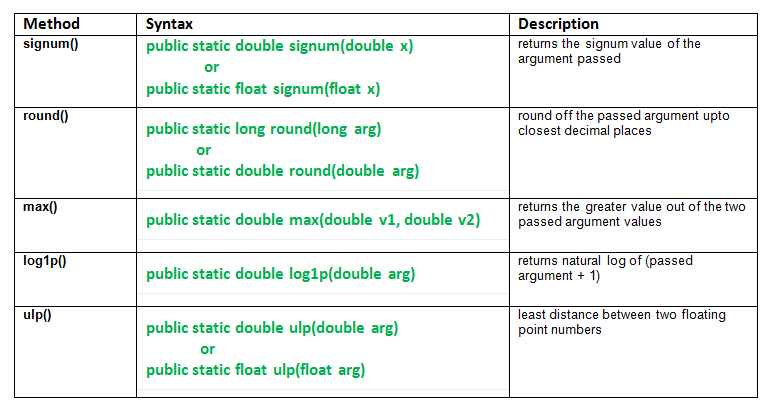Open in App
Not now

# java.math class and its methods | Set 1

• Last Updated : 06 Oct, 2021

Math class provides mathematical functions to perform basic numeric operations such as exponential, logarithm, square root, and trigonometric functions. cosh, sin, tan, abs, bitLength, multiply and many more. Implementations of the functions of Math class do not return bit-for-bit same results. Hence, perform better implementations.

Class Declaration

`public final class Math  extends Object`

This set explains following methods:Methods

1. signum() : java.math.signum() method returns the signum value of the argument passed.
```                                    -1    if x < 0
signum fun(x) =  0    if x = 0
1    if x > 0
```

Note:

Result is NaN, if passed the argument is NaN.;

Syntax:

```public static double signum(double x)
or
public static float signum(float x)
Parameters:
x - the argument whose signum value we need
Returns:
signum value of x
```
2. round() : java.math.round() method round off the passed argument upto closest decimal places.
Note: Result is 0, if the argument is NaN.
Syntax:

```public static long round(long arg)
or
public static double round(double arg)
Parameters:
arg - argument needs to round off
Returns:
round off value of the argument
```
3. max() : java.math.max(double v1, double v2) method returns the greater value out of the two passed argument values.
This method just compares using magnitude without considering any sign.
Syntax:

```public static double max(double v1, double v2)
Parameters:
v1 - first value
v2 - second value
Returns:
v1 or v2 based on which number is greater.
It can return either of the two if v1 = v2.
```

Java code explaining signum(), round(), max() method in Math class.

 `// Java code explaining the Math Class methods``// signum(), round(), max()``import` `java.lang.*;``public` `class` `NewClass``{``    ``public` `static` `void` `main(String args[])``    ``{``        ``// Use of signum() method``        ``double` `x = ``10.4556``, y = -``23.34789``;``        ``double` `signm = Math.signum(x);``        ``System.out.println(``"Signum of 10.45  = "` `+ signm);`` ` `        ``signm = Math.signum(y);``        ``System.out.println(``"Signum of -23.34 = "` `+ signm);``        ``System.out.println(``""``);`` ` `        ``// Use of round() method``        ``double` `r1 = Math.round(x);``        ``System.out.println(``"Round off 10.4556  = "` `+ r1);`` ` `        ``double` `r2 = Math.round(y);``        ``System.out.println(``"Round off 23.34789 = "` `+ r2);``        ``System.out.println(``""``);`` ` `        ``// Use of max() method on r1 and r2``        ``double` `m = Math.max(r1, r2);``        ``System.out.println(``"Max b/w r1 and r2 = "` `+ r2);``    ``}``}`

Output:

```Signum of 10.45  = 1.0
Signum of -23.34 = -1.0

Round off 10.4556  = 10.0
Round off 23.34789 = -23.0

Max b/w r1 and r2 = -23.0
```
1. log1p() : java.math.log1p() method returns natural log of (passed argument + 1).
Syntax:

```public static double log1p(double arg)
Parameters:
arg - the argument
Returns:
log of (argument + 1).
This result is within 1 unit in the last place of exact result.
```
2. ulp() : java.math.ulp() method returns Unit of least precision(ulp) ie. the least distance between two floating point numbers.
Here, it is the least distance b/w the argument and next larger value.
Syntax:

```public static double ulp(double arg)
or
public static float ulp(float arg)
Parameters:
arg - argument passed.
Returns:
least distance b/w the argument and next larger value.
```

Java code explaining ulp(), log1p() method in Math class.

 `// Java code explaining the Math Class methods``// ulp(), log1p()``import` `java.lang.*;``public` `class` `NewClass``{``    ``public` `static` `void` `main(String args[])``    ``{``        ``// Use of ulp() method``        ``double` `x = ``34.652``, y = -``23.34789``;``        ``double` `u = Math.ulp(x);``        ``System.out.println(``"ulp of 34.652    : "` `+ u);`` ` `        ``u = Math.ulp(y);``        ``System.out.println(``"ulp of -23.34789 : "` `+ u);``        ``System.out.println(``""``);`` ` `        ``// Use of log() method``        ``double` `l = ``99``;``        ``double` `l1 = Math.log1p(l);``        ``System.out.println(``"Log of (1 + 99)  : "` `+ l1);`` ` `        ``l1 = Math.log(``100``);``        ``System.out.println(``"Log of 100       : "` `+ l1);``    ``}``}`

Output:

```ulp of 34.652    : 7.105427357601002E-15
ulp of -23.34789 : 3.552713678800501E-15

Log of (1 + 99)  : 4.605170185988092
Log of 100       : 4.605170185988092
```

This article is contributed by Mohit Gupta. If you like GeeksforGeeks and would like to contribute, you can also write an article using write.geeksforgeeks.org or mail your article to review-team@geeksforgeeks.org. See your article appearing on the GeeksforGeeks main page and help other Geeks.